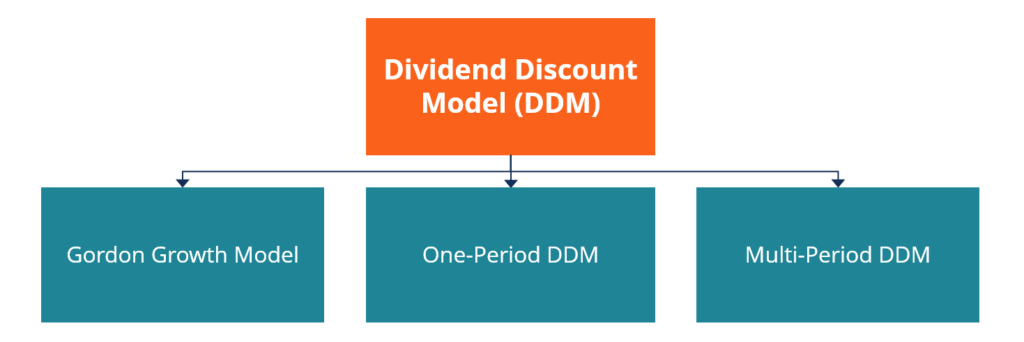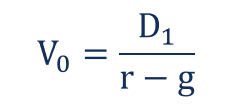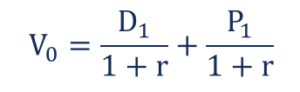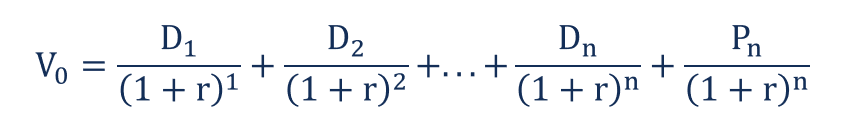# Dividend Discount Model

Assumes that the current fair price of a stock equals the sum of all company’s future dividends discounted back to their present value

## What is the Dividend Discount Model?

The Dividend Discount Model (DDM) is a quantitative method of valuing a company’s stock price based on the assumption that the current fair price of a stock equals the sum of all of the company’s future dividends discounted back to their present value.### Breaking Down the Dividend Discount Model

The dividend discount model was developed under the assumption that the intrinsic value of a stock reflects the present value of all future cash flows generated by a security. At the same time, dividends are essentially the positive cash flows generated by a company and distributed to the shareholders.

Generally, the dividend discount model provides an easy way to calculate a fair stock price from a mathematical perspective with minimum input variables required. However, the model relies on several assumptions that cannot be easily forecasted.

Depending on the variation of the dividend discount model, an analyst requires forecasting future dividend payments, the growth of dividend payments, and the cost of equity capital. Forecasting all the variables precisely is almost impossible. Thus, in many cases, the theoretical fair stock price is far from reality.

### Formula for the Dividend Discount Model

The dividend discount model can take several variations depending on the stated assumptions. The variations include the following:

#### 1. Gordon Growth Model

The Gordon Growth Model (GGM) is one of the most commonly used variations of the dividend discount model. The model is called after American economist Myron J. Gordon, who proposed the variation.

The GGM is based on the assumptions that the stream of future dividends will grow at some constant rate in future for an infinite time. Mathematically, the model is expressed in the following way:Where:

• V– the current fair value of a stock
• D– the dividend payment in one period from now
• r – the estimated cost of equity capital (usually calculated using CAPM)
• g – the constant growth rate of the company’s dividends for an infinite time

#### 2. One-period Dividend Discount Model

The one-period discount dividend model is used much less frequently than the Gordon Growth model. The former is applied when an investor wants to determine the intrinsic price of a stock that he or she will sell in one period from now. The one-period dividend discount model uses the following equation:Where:

• V– the current fair value of a stock
• D– the dividend payment in one period from now
• P– the stock price in one period from now
• r – the estimated cost of equity capital

#### 3. Multi-period Dividend Discount Model

The multi-period dividend discount model is an extension of the one-period dividend discount model wherein an investor expects to hold a stock for the multiple periods. The main challenge of the multi-period model variation is that forecasting dividend payments for different periods is required. The model’s mathematical formula is below:CFI is the official provider of the global Financial Modeling & Valuation Analyst (FMVA)™ certification program, designed to help anyone become a world-class financial analyst. To keep advancing your career, the additional resources below will be useful:

• Capital Gains Yield
• Dividend Per Share
• Ex-Dividend Date
• Stock Option

### Valuation Techniques

Learn the most important valuation techniques in CFI’s Business Valuation course!

Step by step instruction on how the professionals on Wall Street value a company.

Learn valuation the easy way with templates and step by step instruction!